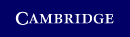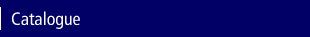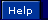Home > Catalogue > Materials ScienceSearch this book

Details

• Page extent: 252 pages
• Size: 253 x 177 mm
• Weight: 0.66 kg

Hardback

(ISBN-13: 9780521867054)

• Also available in Paperback | Adobe eBook
• For price and ordering options, inspection copy requests, and reading lists please select:

Please note local prices may applyView Excerpt as PDF (277KB)

1 Microstructural Analysis

Many properties of materials depend on the grain size and the shape of grains. Analysis of microstructures involves interpreting two-dimensional cuts through three-dimensional bodies. Of interest are the size and aspect ratios of grains, and the relations between grain size and the amount of grain boundary area per volume. Also of interest is the relation between the number of faces, edges, and corners of grains.

Grain size

There are two commonly used ways of characterizing the grain size of a crystalline solid. One is the ASTM grain size number, N, defined by

 n = 2N-1   or   N = 1 + ln(n)/ln 2, (1.1)

where n is the number of grains per square inch observed at a magnification of 100X. Large values of N indicate a fine grain size. With an increase of the grain diameter by a factor of √2, the value of n is cut in half and N is decreased by 1.

EXAMPLE 1.1. Figure 1.1 is a micrograph taken at 200X. What is the ASTM grain size number?

1.1. Counting grains in a microstructure at 200X.

 Image not available in HTML version

SOLUTION: There are 29 grains entirely within the micrograph. Counting each grain on an edge as one half, there are 22/2 = 11 edge grains. Counting each corner grain as one quarter, there is 1 corner grain. The total number of grains is 41. The 12 square inches at 200X would be 3 square inches at 100X, so n = 41/3 = 13.7. From Equation (1.1),

 N = ln(n)/ln(2) + 1 = 4.78 or 5.

The average linear intercept diameter is the other common way to characterize grain sizes. The system is to lay down random lines on the microstructure and count the number of intersections per length of line. The average intercept diameter is then ℓ̅ = L/N, where L is the total length of line and N is the number of intercepts. Alternatively, a rectangular grid of lines may be laid down on an equiaxed microstructure.

EXAMPLE 1.2. Find the average intercept diameter for the micrograph in Figure 1.1.

SOLUTION: In Figure 1.2, 6 x 4 + 5 x 3 = 39 inches of line are superimposed on the microstructure. This corresponds to (39 in. /200)(25.4mm/in.) = 4.95mm. There are 91 intercepts so ℓ̅ = .495/91 = 0.054mm = 54μm.

1.2. Finding the linear intercept grain size of a microstructure at 200X.

 Image not available in HTML version

For random microstructures, ℓ̅ and the ASTM grain size are related. An approximate relationship can be found by assuming that the grains can be approximated by circles of radius, r. The area of a circular grain, πr2, can be expressed as the average linear intercept, ℓ̅, times its width, 2r, as shown in Figure 1.3, so ℓ̅ · 2r = πr2. Therefore,

 r = (2/π)ℓ̅   or   ℓ̅ = (π/2)r (1.2)

1.3. The area of a circle, πr2, equals the average intercept times twice the radius, 2ℓ̅r, so ℓ̅ = (π/2)r.

 Image not available in HTML version

Thus, the area per grain is A = 2r ℓ̅ = (4/π)ℓ̅2. The number of grains per area is (π/4)/ℓ̅2. From the definition of n, the number of grains per area is also n[(25.4mm/in.)/(100in.)]2. Substituting n = 2N-1 = 2N/2 and equating,

 (π/4)/ℓ̅2 = (2N/2)(0.254)2. (1.3)

Solving for ℓ̅,

 ℓ̅ = 4.93/2N/2. (1.4)

Often grains are not equiaxed. They may be elongated in the direction of prior working. Restriction of grain growth by second-phase particles may prevent formation of equiaxed grains by recrystallization. In these cases, the linear intercept grain size should be determined from randomly oriented lines or an average of two perpendicular sets of lines. The degree of shape anisotropy can be characterized by an aspect ratio, α, defined as the ratio of average intercept in the direction of elongation to that at 90°:

 α = ℓ̅∥/ℓ̅⊥. (1.5)

Relation of grain boundary area per volume to grain size

The grain boundary area per volume is related to the linear intercept. Assuming that grain shapes can be approximated by spheres, the grain boundary surface per grain is 2πR2, where R is the radius of the sphere. (The reason that it is not 4πR2 is that each grain boundary is shared by two neighboring grains.) The volume per spherical grain is (4/3)πR3, so the grain boundary area/volume, Sv, is given by

 Sv = (2πR2)/[(4/3)πR3] = 3/(2R). (1.6)

To relate the spherical radius, R, to the linear intercept, ℓ̅, consider the circle through its center, which has an area of πR2 (Figure 1.4). The volume equals the product of this area, πR2, and the average length of line, ℓ̅, perpendicular to it, v = ℓ̅πR2. Therefore, (4/3)πR3 = πR2ℓ̅ or R = (3/4)ℓ̅. Substituting into Sv = 3/(2R),

 Sv = 2/ℓ̅. (1.7)

1.4. The volume of a sphere = ℓ̅πR2.

 Image not available in HTML version

Relation of intersections per area and line length

The number of intersections per area of dislocations with a surface is less than the total length of dislocation line per volume. Consider a single line of length L in a box of height h and area of A. The number of intersections per area, NA, equals 1/A (Figure 1.5). The length per volume is LV = L/(hA) so NA/LV = h/L. Because cos θ = h/L, NA/LV = cos θ. For randomly oriented lines, the number oriented between θ and θ + dθ is dn = ndf, where df = sin θdθ. For randomly oriented lines, NA/LV = ∫ cos θ sin θdθ = 1/2. Therefore,

 NA = LV/2. (1.8)

1.5. Relation of the number of intersections per area with the length of line per volume.

 Image not available in HTML version

Volume fraction of phases

Point counting is the easiest way of determining the volume fraction of two or more phases in a microstructure. The volume fraction of a phase equals the fraction of points in an array that lies on that phase. A line count is another way of finding the volume fraction. If a series of lines are laid on a microstructure, the volume fraction of a phase equals the fraction of the total line length that lies on that phase.

Alloy composition from volume fraction of two or more phases

The composition of an alloy can be found from the volume fractions of phases. The relative weight of component B in the α phase is (Vα)(ρα)(Cα), where Vα is the volume fraction of α, ρα is the density of α, and Cα is the composition (%B) of the α phase. With similar expressions for the other phases, the relative weight of component B, WB, is given by

 WB = (Vα)(ρα)(Cα) + (Vβ)(ρβ)(Cβ) + ··· (1.9)

With similar expressions for the other components, the overall composition of the alloy is

 %B = 100WB /(WA + WB + ···). (1.10)

Microstructural relationships

Microstructures consist of three-dimensional networks of cells or grains that fill space. Each cell is a polyhedron with faces, edges, and corners. Their shapes are strongly influenced by surface tension. However, before examining the nature of three-dimensional microstructures, the characteristics of two-dimensional networks will be treated.

A two-dimensional network of cells consists of polygons, edges (sides), and corners. The number of each is governed by the simple relation

 P - E + C = 1, (1.11)

where P is the number of polygons, E is the number of edges, and C is the number of corners. Figure 1.6 illustrates this relationship. If the microstructure is such that three and only three edges meet at each corner, E = (3/2)C, so

 P - C/2 = 1 and P - E/3 = 1. (1.12)

1.6. Three networks of cells illustrating that P - E + C = 1.

 Image not available in HTML version

For large numbers of cells, the one on the right-hand side of Equations (1.9) and (1.10) becomes negligible, so E = 3P and C = 2P. This restriction of three edges meeting at a corner also requires that the average angle at which the edges meet is 120° and that the average number of sides per polygon is six.

If the edges were characterized by a line tension (in analogy to the surface tension of surfaces in three dimensions) and if the line tensions for all edges were equal, equilibrium would require that all edges meet at 120°, so cells with more than six edges would have to be curved with the center of curvature away from the cell and those cells with fewer than six sides would be curved the opposite way, as shown in Figure 1.7. Since boundaries tend to move toward their centers of curvature, the cells with large numbers of sides would tend to grow and those with few sides should shrink. Only a network in which all of the cells were regular hexagons would be stable.

1.7. The sides of grains with fewer than six neighbors are inwardly concave (left). The sides of grains with more than six neighbors are outwardly concave (right).

 Image not available in HTML version

Three-dimensional relations

Euler proposed that for a single body

 C - E + F - B = 1. (1.13)

For an infinite array of three-dimensional bodies,

 C - E + F - B = 0. (1.14)

Here, B is the number of bodies (grains), F is the number of faces, E is the number of edges, and C is the number of corners. Consider an isolated cube, for example. There is one body, and there are six faces, 12 edges, and eight corners. B = 1, F = 6, E = 12, and C = 8. 8 - 12 + 6 - 1 = 1. For an infinite array of stacked cubes, each face is shared by two cubes so F = 6B/2. Each edge is shared by four cubes so E = 8B/4, and each corner is shared by eight cubes so C = 12B/8. Substituting into Euler’s equation, 8B/8 - 12B/4 + 6B/2 - B = 0. Table 1.1 illustrates Equation (1.11) for several simple polyhedra.

Polyhedron Faces (F) Edges (E) Corners (C) F − E + C Tetrahedron 4 6 4 2 Cube 6 12 8 2 Octahedron 8 12 6 2 Dodecahedron(cubic) 12 24 14 2 Dodecahedron(pentag.) 12 30 20 2 Tetrakaidecahedron 14 36 24 2

Kelvin tetrakaidecahedron

Grains in a real material have certain restrictions: Each corner is shared by four grains, and each edge is shared by three grains. Furthermore, grains stack in such a way as to fill space. Very few simple shapes fulfill these conditions. One simple shape is the tetrakaidecahedron proposed by Lord Kelvin.* Figure 1.8 shows that it can be thought of as a cube with each corner truncated by an octahedron. Alternatively, it can be thought of as an octahedron with each corner truncated by a cube. There are 14 faces, 36 edges, and 24 corners. For an infinite array of these polyhedra,

 F = 14B/2 = 7B, C = 24B/4 = 6B, and E = 36B/3 = 12B, so C - E + F - B = 6B - 12B + 7B - B = 0.

1.8. The Kelvin tetrakaidecahedron and its construction by truncation of an octahedron by a cube. The edges of the tetrakaidecahedron are one third as long as the edges of the octahedron.

 Image not available in HTML version

This shape is a useful approximation for analyzing grains in a polycrystal. For example, calculation of the surface area of the faces to the grain volume can be compared with other solid shapes and a sphere. Six of these are squares parallel to {100} planes and eight are regular hexagons parallel to {111} planes. There are 24 corners and 36 edges. Thus, the total length of edges is 36e, where e is the length of an edge, and the total surface area is the area of the six square faces plus the eight hexagonal faces:

 6e2 + 8(3√3)e2 = 47.569e2.

The volume is the volume of the octahedron less the volume of the six truncated pyramids:

 [9√2 - 6(1/3√2)]e3 = 8√2e3 = 11.314e3.

Of the 14 faces, 6 have four edges and 8 have six edges. The average number of edges per face is (6 × 4 + 8 × 6)/14 = 51/7 This is very close to the results of experiments on β brass, vegetable cells, and soap bubbles, as shown in Figure 1.9. For the Kelvin tetrakaidecahedron the ratio of surface area to that of a sphere of the same volume is 1.099. Most other shapes have much higher ratios.

1.9. Frequency of polygonal faces with different numbers of edges. Data from C. S. Smith, in Metal Interfaces (Cleveland, OH: ASM, 1952). Reprinted with permission from ASM International®. All rights reserved. www.asminternational.org.

 Image not available in HTML version

NOTES OF INTEREST

1. Lord Kelvin (1824–1907), a Scottish mathematician and physicist, did the pioneering work on the second law of thermodynamics, arguing that it was the explanation of irreversible processes. He noted that the continual increase of entropy would lead to a universe with a uniform temperature and maximum entropy.
2. Waire and Phelan* report that space filling is 0.3% more efficient with an array of of six polyhedra with 14 faces and two polyhedra with 12 faces than with the Kelvin tetrakaidecahedron. (This calculation allows faces in each to be curved.) The 14-faced polyhedra have 12 pentagonal and 2 hexagonal faces, while the 12-faced polyhedra have distorted pentagons for faces. The average number of faces per polyhedra = (6 × 14 + 2 × 12)/8 = 13.5.

PROBLEMS

 1. A soccer ball has 32 faces. They are all either pentagons or hexagons. How many are pentagons? 2. Figure 1.10 is a microstructure at a magnification of 200X. A. Determine the ASTM grain size number. B. Determine the intercept grain size. C. Compare the answers to A and B using Equation (1.5). 3. Count the number of triple points in Figure 1.10 and deduce the ASTM grain size from this count. Compare with your answer to Problem 2A.

1.10. Hypothetical microstructure at a magnification of 200Ⅹ.

 Image not available in HTML version

 4 What is the linear intercept grain size (in millimeters) corresponding to an ASTM grain size number of 8? 5 Dislocation density is often determined by counting the number of dislocations per area intersecting a polished surface. If the dislocation density in cold-worked copper is found to be 2 × 1010/cm2, what is the total length of dislocation line per volume? 6 Calculate the average number of edges per face for the space-filling array of polyhedra reported by Waire and Phelan. 7 If the ASTM grain size number is increased by one, by what factor is the number of grains per volume changed? 8 If a material with grains shaped like tetrakaidecahedra were recrystallized and new grains were nucleated at each corner, by what factor would the grain diameter, ℓ̅, change? 9 Derive an equation relating the aspect ratio of a microstructure after uniaxial tension to the strain, assuming that the microstructure was initially equiaxed. 10 Determine the aspect ratio in Figure 1.11.

1.11. Microstructure for Problem 10.

 Image not available in HTML version

 11 Determine the volume fraction of graphite in the cast iron shown in Figure 1.12.

1.12. A schematic drawing of ferritic ductile cast iron. The white areas are ferrite and the dark circles are graphite.

 Image not available in HTML version